## Wednesday, September 24, 2008

### Window Patterns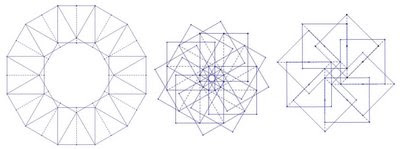Start with a square piece of paper (like a Post it note), fold and unfold it in half along a mid-line or along a diagonal. Take another identical square, and fold and unfold it the same way. Decide on some way to place the second square on the first, so that the second is somewhat rotated. Use only the edges of the square and the creases that you made to determine the placement. Make your placement precise so that your "rule" can be described exactly in terms of the edges and creases. Repeat this process, placing a third square on top of your second square using exactly the same rule. Repeat until your placing of papers leads you back to the first piece.The resulting construction might look something like the one shown on the left below. If you take your papers, set them in place with some careful light gluing, and place them on a window, the sunlight passing through the overlapping papers creates a stained-glass effect that shows a variety of shapes.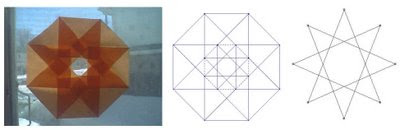This sort of construction is a simplified version of what William Gibbs describes in his book "Window Patterns." In Gibbs' treatment, the pattern is partially planned in advance, and then the dimensions of the rectangular pieces of paper that make up the pattern are determined using a little trigonometry. This process can be simplified by starting with a more limited range of options for paper dimension and placement. It turns out that a surprising number of window patterns can be created by only using squares, their mid-lines, and their diagonals, and that these patterns invariably have "special triangles" and related regular polygons and star-polygons embedded within them.

Here are a two more "placement rules" and the patterns that they give rise to.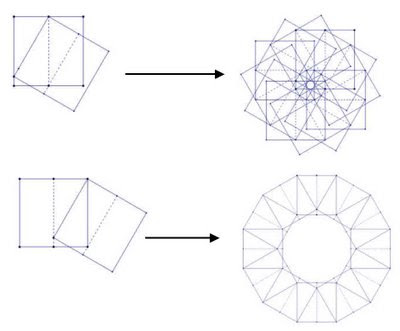The diagrams were created using Geometer's Sketchpad - if you construct the rule using translations applied to a constructed square, you can use the iteration feature to create the final pattern. GSP provides a good environment for planning out the patterns prior to constructing them with paper, and building the plans in GSP is enjoyable and instructive as well.

## Friday, September 19, 2008

### Star Polygons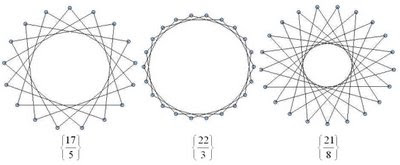Starting with p (p a positive integer) equally distributed dots (vertices) around a circle. Connecting each dot to the next as you move around the circle will give you a regular p-gon - a p-sided polygon with p vertices. If, however, instead of connecting each dot to the one next to it you skip over a fixed number of dots, then you might end up with a star-like pattern, like the ones shown above. In this process, imagine that you start with a particular vertex and move in a counter-clockwise direction. If there are any dots left-over when you get back to the dot you started from, just throw away the unconnected dots.

The Schläfli notation for polygons is very useful for describing regular connected star polygons, and provides an example of how sometimes calculations with notation match exactly with calculations done with diagrams. In this notation, regular polygons like triangle, square, pentagon, etc. are written as {3}, {4}, and {5} respectively. A regular p-gon is written as {p}. If when drawing your p-gon you connect to the second next vertex instead of the first, then you would write this as {p/2}. If you connect to the q'th next vertex, then you would write this polygon as {p/q}. Note that if you are just connecting to the next vertex to make a regular p-gon, this notation gives you {p/1} = {p}, as you would expect.

If you start playing with this process you will notice that {p/q} gives you the same polygon as {p/(p-q)} (as long as you ignore the orientation of the polygon). You may also notice that if q is larger than p, you end up repeating the same patterns, in particular {p/q} = {p/(q mod p)}. Also you will notice that if p and q have common factors, you end up having skipped vertices. In our process we are throwing these away to ensure that our polygons are connected, but you can extend the process and keep them (see note below).

The process described is straightforward to implement in a program. The images shown here were generated in Tinkerplots. To implement it in Tinkerplots, you need two sliders - p and q, and the following attributes:

n = caseIndex()
theta = 2*n*pi(1-q/p)
x = cos(theta)
y = sin(theta)

If you create a plot with y vertical and x horizontal, choose "show connecting lines" and add a filter n<=p+1, you can add a large number of cases to the collection (~200, say) and be able to slide p and q to create a wide variety of connected star polygons. The only restriction is that p must be less than the number of cases you have created. There is nothing special about using Tinkerplots here - any programming environment with reasonable graphics should do a reasonable job (Logo would be fine. :)).

The polygons below are the regular connected polygons based on 12 vertices. Because 12 is divisible by 2, 3, 4, and 6 we end up with regular polygons triangle {3}, square {4}, hexagon {6} and only one star polygon {12/5}. The "degenerate" polygon {2} is known as a "digon." Here, drawing the diagram first and then seeing what polygon comes out will give you the same result as dividing p/q first and then drawing the corresponding polygon. In this sense, the notation and diagrams nicely reflect each other.Contrast this with the family of star polygons that are generated when a prime number of vertices are used. The images below are the family of regular connected polygons generated on 13 vertices.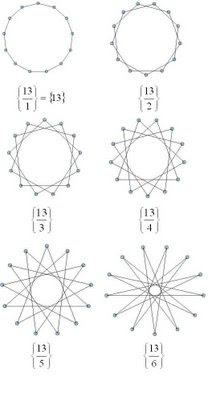Note - by throwing away the unconnected dots in our process we are ignoring star polygons that are made of overlapping disjoint star or regular polygons, for example two overlapping triangles that make a star of David. These also work well with the Schläfli notation. To create these overlapping polygons, if you have any skipped vertices, you just begin your process again beginning with one of the vertices you skipped over. In the case of {6/2}, instead of getting one triangle {3} you will get two overlapping triangles, or 2{3}. To write a program that would draw these you would want to use something more sophisticated than Tinkerplots.

## Tuesday, September 16, 2008

### Extended Multiplication TablesA surprisingly interesting structure is the extended multiplication table, shown above for the numbers seven to ten. The algorithm for drawing these is straight forward - for an n-extended table, start out as if you were writing a "regular" multiplication table, but extend each row so that it gets as close to, without exceeding, n. Another way to think about it is to write out rows of "skip counting up to n" by i for integers i from 1 to n.

This is called an extended multiplication table since it contains a "traditional" multiplication table inside it. The 12-extended table below contains a traditional 3x3 multiplication table.It turns out that 1 appears in an extended table once, and prime numbers appear exactly twice (once in the first column, and once in the first row). In general, for a natural number n, how many times does n appear in the n-extended table?

Before looking at that question, you might want to think about finding easier ways to draw the tables. Drawing out these tables by hand can be tedious - a simple program or spreadsheet might be easier. You can use Fathom, for example, to create the table data and draw it in the collections display. Create a slider m and the attributes listed in the table below (click on the image to see a larger version).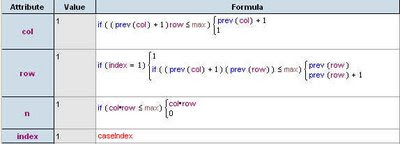Modify the collection display attributes to draw the tables in the collection box. By adding lots of cases and using the slider m to filter out the ones you don't need, you can vary the size of the table easily.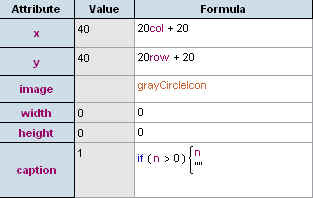Interestingly, the answer to the question "how many times does n appear in the n-extended table?" is the same as the answer to the question posed in a previous post about factor lattices.

# of occurrances of n in the n-extended table = # of nodes in the factor lattice Fn

You can also recast both of these questions (how many occurances of n in the n-extended table, and how many nodesin the Fn factor lattice) as a combinatorial "balls in urns" problem.

Consider a set of colored balls where there are m different colours, where there are ki balls of color i, where i ranges from 1 to m. This would give a total number of balls equal to k1+k2+...+km. Suppose you were to distribute these balls in two urns. How many different distributions would there be? Using some counting techniques, you will find that the answer is (k1+1)*(k2+1)*...*(km+1).

How is this connected to the other problems? Consider the prime factorization of the number. For each prime, choose a colour, and for each occurance of the prime in the factorization, add a new ball of that color. For example for 12 = 3*3*2, choose two colours - say blue=3 and red=2. Since 3 occurs twice and 2 occurs once, there should be two blue balls and one red ball. Now consider distributing these balls in two urns. It turns out that you get (2+1)*(1+1) = 6 possibilities. This is the same number of times 12 occurs in the 12-extended table, and the same number of nodes in the 12-factor lattice. The image below shows the 12-extended table, the 12-factor lattice, and the "ball and urn problem" for the numer 12.For a number n with the prime factorization:The answer to all three questions is given by: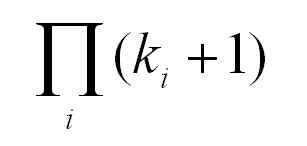## Wednesday, September 10, 2008

### Phyllotaxis Spirals 2The previous post showed some phyllotaxis-like spirals that were created using TinkerPlots. If you bring the same file into Fathom, you can use its support for collection display to create a "graduated" picture that makes the more central seeds look smaller (younger) than the more mature outer seeds.

If you have already created the phyllotaxis data in Fathom, or opened the data created in TinkerPlots in Fathom, you can change the display you get when you drag open a collection box. Under the display tab on the Collection Inspector in Fathom you can set the display properties so that cases no longer show up as uniform gold balls. Setting the display attributes to the values below should give you a growing spiral like the one pictured above.

x = 10x+400
y = 10y+400
image = greyCircleIcon

width = sqrt(r*10)
height = sqrt(r*10)
caption=""

Note tha the x and y that appear on the right-hand side of the equations above are the x and y attributes that you defined for the data, and the x and y that appear on the left-hand correspond to the position of the icons. You can experiment with other formulas for width and height - using a slider to provide a variable instead of the number "10" gives more flexibility.

The images below show some of the other spirals you can obtain by varying the angle between the seeds, as mentioned in the previous post.## Friday, September 5, 2008

### Phyllotaxis SpiralsPhyllotaxis is a term used for the patterns that emerge in the growth of plants. Spiral phyllotaxis is observed in the heads of sunflowers, in pine-cones and pineapples, and in a variety of other plants.

Phyllotaxis is a popular topic in mathematics recreation - it's interesting in its own right and also related to other perennial favorites, Fibonacci numbers and the golden ratio.

The article Modeling Spiral Growth in a GSP Environment describes how to model phyllotaxis-like patterns in GSP. Although GSP works reasonably well, TinkerPlots or Fathom environments seem to be better suited to capturing this particular model - they make the formulas more explicit and easy to manipulate, and they allow for the data generated to be viewed in a variety of ways. The images above were created by porting this model to TinkerPlots.

As the article suggests, experimenting with the the angle between successive seeds allows you to see different resulting patterns - angles that are multiples of rational numbers create patterns of rays while irrational numbers (actually approximate values) give spirals, or spiral/ray combinations (the rays form as the approximation gets more "rational"). A good choice for approximating actual phyllotaxis patterns is to use tau = (1+sqrt(5))/2 in your angle. Here is a listing for the attributes required to generate the pattern in TP or Fathom. The graph/plot is simply the x attribute on the horizontal and the y attribute on the vertical (in TP these need to be fully separated).

n = caseIndex
base_angle = pi*(1+sqrt(5))
r = sqrt(n)

theta = n*base_angle

x = r*cos(theta)
y = r*sin(theta)

The images shown in this post use a collection of 500 cases or "seeds". The base angle is 2pi*tau, and the actual angle for a given seed is a multiple of this base angle.

The model is nice looking and easy to build, but it models only the end result of the growth process, not the process itself. It winds new seeds around the outer edge based on a pre-determined angle. A better model would be one that mirrors what is understood to be the underlying phonomena - new seeds are added to the center and old seeds are pushed out following a set of rules. Under this dynamic method, the angles and spirals are an emergent aspect of the system, rather than the explicit result. This website describes how such a dynamical system could be modeled.

Although the Fathom/TP model does not model the dynamical system that underlies phyllotaxis, it's fun to play with in its own right. You can manually alter the base_angle attribute as suggested by the GSP article. If you add a parameter (a slider) to help you vary the angle, you can obtain a whole family of spiral/ray patterns whose properties you could take a closer look at. Different combinations of angles and sliders will give you various levels of control over the image.

For example, change the formula for base_angle to base_angle = pi*(1+sqrt(5))*base, and create a slider "base". The image below shows the spirals obtained for base = 1...6.Update: Here is an example of how to draw spirals like this in R.

### Factor LatticesThe objects pictured above are interesting structures - they are derived from the prime factorization of a given number n. They can be described in a number of ways - for example, as directed graphs. Because they are nicely structured, they actually form something more special - a lattice. Accordingly, these structures are called factor lattices.

It's easy to start drawing these by hand following the instructions below.

1. The first node is 1
2. Draw arrows out of this node for each of the prime factors of n.
3. The arrows that you just drew should connect to nodes labled with the prime factors of n.

Now, for each of the new nodes that you drew do the following:

4. Start from a node x that is not equal to n.
5. Draw arrows out of this node for each of the prime factors of n/x.
6. The arrows that you just drew (one for each p = n/x) should connect to nodes labled with the numbers p*x.

7. Now repeat 4,5, and 6 for each new node that you have drawn that is not equal to n.

This process is recursive, and ends when you have the complete lattice. The process is well suited for implementation as a computer program - the images above were created using SAGE using output from a Java program based on the algorithm above.

Manually trying out the steps out for a number like n = 24 goes something like this: First write out the prime factorization of 24, 24=(2*2*2)*3 = (2^3)*3. Starting with 1, draw arrows out to 2 and 3. Now looking at each node and following the algorithm, from the 2 you will get arrows out to 4 and 6. From the 3 you will get an arrow out to 6 as well. From 4 you will get arrows out to 8 or 12. From 6 you will get an arrow out to 12 as well. From 8 and from 12 you get arrows out to 24, and you are done.

In general, the algorithm produces a lattice that can be described as follows. Each node is a factor of the given number n. Two nodes are connected by an edge if their prime factorization differs by a single prime number. In other words, if a and b are nodes, and p = b/a, then there is an arrow p:a-->b.

It's a good exercise to make the connections between the lattice structure and the prime factorization of a number n.

1. What does the factor lattice of a prime number look like?
2. If a number is just a power of a prime, what does its lattice look like?
3. If you know the factorization, can you find the number of nodes without drawing the lattice.

The answer to the last question (3) can be expressed as:For example, if n = 24= 2^3*3, then the number of nodes will be (3+1)(1+1) = 8

That these structures can be thought of as "lattices"comes from the fact that you can think of the arrows as an ordering of the nodes, ab. The number 1 is always the least node in the factor lattice for n, while n itself is the greatest node. The property that actually makes these structures a "lattice" is that for any two nodes there is always a lower-bound for any pair of nodes in the lattice, and always an upper-bound for the pair (these are often referred to as meets and joins).

The Wolfram Demonstrations Project has a nice factor lattice demo that will draw factor lattices for a large number of integers for you. There is also a good Wikipedia entry for lattices in general.